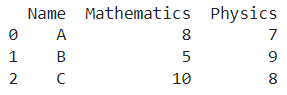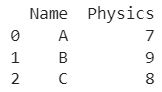# Convert List Of Dictionaries To A Pandas DataFrame

A DataFrame is the primary data structure of the Pandas library in Python and is commonly used for storing and working with tabular data.

A common operation that could be performed on such data is to convert a list of dictionaries to a pandas dataframe in order to accumulate the information present in different dictionaries.

To start working with Pandas, we first need to import it:

``import pandas as pd``

## Running Example

Let us understand this operation with the help of an example. Consider the following DataFrame containing 3 students with names A, B, and C and their corresponding marks (out of 10) for two subjects, Mathematics and Physics.Now, let's say we have each row of this required dataframe present in the form of a dictionary with us with the column labels i.e. Name, Mathematics, and Physics being the keys and with corresponding values for each row.

These dictionaries are present in a list assigned to a variable named a. The code and corresponding output for such a list is as follows:

``````# List of row-wise dictionaries

a = [{'Name' : 'A', 'Mathematics' : 8, 'Physics' : 7}, {'Name' : 'B', 'Mathematics' : 5, 'Physics' : 9}, {'Name' : 'C', 'Mathematics' : 10, 'Physics' : 8}]

# Printing
print(a)``````

Output

[{'Name': 'A', 'Mathematics': 8, 'Physics': 7}, {'Name': 'B', 'Mathematics': 5, 'Physics': 9}, {'Name': 'C', 'Mathematics': 10, 'Physics': 8}]

Let us look at different ways of performing this operation of creating a dataframe from such a list of dictionaries on a given DataFrame :

## 1. Using the DataFrame() function

In this method, we use the DataFrame() function to convert a list of dictionaries to a pandas dataframe. The desired list here is as specified earlier and stored in a variable named a.

This variable is passed as a parameter to the dataframe() function that returns a dataframe object. This returned dataframe object is assigned to a variable named df which is later printed.

Let us take a look at the corresponding code snippet and generated output for this method :

``````# Import pandas
import pandas as pd

# List of row-wise dictionaries
a = [{'Name' : 'A', 'Mathematics' : 8, 'Physics' : 7}, {'Name' : 'B', 'Mathematics' : 5, 'Physics' : 9}, {'Name' : 'C', 'Mathematics' : 10, 'Physics' : 8}]

# Creating the dataframe
df = pd.DataFrame(a)

# Printing
print(df)``````

Output:## 2. Using the dataframe.from_dict() function

In this method, we use the DataFrame.from_dict() function to convert a list of dictionaries to a pandas dataframe. The desired list here is as specified earlier and stored in a variable named a.

This variable is passed as a parameter to the dataframe.from_dict() function that returns a dataframe object. This returned dataframe object is assigned to a variable named df which is later printed.

Let us take a look at the corresponding code snippet and generated output for this method:

``````# Import pandas
import pandas as pd

# List of row-wise dictionaries
a = [{'Name' : 'A', 'Mathematics' : 8, 'Physics' : 7}, {'Name' : 'B', 'Mathematics' : 5, 'Physics' : 9}, {'Name' : 'C', 'Mathematics' : 10, 'Physics' : 8}]

# Creating the dataframe
df = pd.DataFrame.from_dict(a)

# Printing
print(df)``````

Output:## 3. Using the dataframe.from_records() function

In this method, we use the DataFrame.from_records() function to convert a list of dictionaries to a pandas dataframe.

The desired list here is as specified earlier and stored in a variable named a. This variable is passed as a parameter to the dataframe.from_records() function that returns a dataframe object.

This returned dataframe object is assigned to a variable named df which is later printed. Let us take a look at the corresponding code snippet and generated output for this method:

``````# Import pandas
import pandas as pd

# List of row-wise dictionaries
a = [{'Name' : 'A', 'Mathematics' : 8, 'Physics' : 7}, {'Name' : 'B', 'Mathematics' : 5, 'Physics' : 9}, {'Name' : 'C', 'Mathematics' : 10, 'Physics' : 8}]

# Creating the dataframe
df = pd.DataFrame.from_records(a)

# Printing
print(df)``````

Output :## 4. Using specific columns

In the earlier methods we considered all the keys that were present in the given list of dictionaries but what if we want to consider only a subset of these keys to create a dataframe, that is, consider only specific columns.

We can use the same functions as we used in the previous examples along with a parameter named columns containing the list of desired column names. Here those column names are Name and Physics.

Let us take a look at the corresponding code snippet and generated output for this method:

``````# Import pandas
import pandas as pd

# List of row-wise dictionaries
a = [{'Name' : 'A', 'Mathematics' : 8, 'Physics' : 7}, {'Name' : 'B', 'Mathematics' : 5, 'Physics' : 9}, {'Name' : 'C', 'Mathematics' : 10, 'Physics' : 8}]

# Creating the dataframe
df = pd.DataFrame(a, columns = ['Name', 'Physics'])

# Printing
print(df)``````

Output :## Conclusion

In this topic, we have learned to convert a list of dictionaries to a Pandas DataFrame, following a running example of test scores of students in different subjects, thus giving us an intuition of how this concept could be applied in the real-world situations. Feel free to reach out to info.javaexercise@gmail.com in case of any suggestions.

Trending